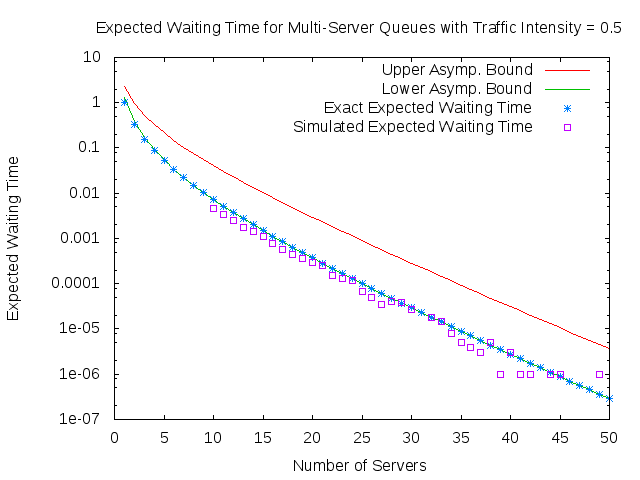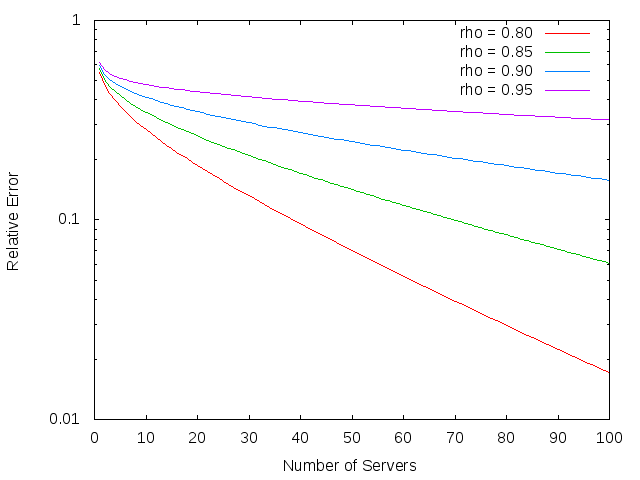# Asymptotic behaviour of M/M/K queue

The M/M/K queue has a central role in many discussion about more general queues. It will therefore be useful to gain a better insight into its behaviour. We have been specifically interested in the behaviour of$\mathbb{E}\left[W^{M/M/K}\right]$ as we increase the number of servers while keeping the traffic intensity,$\rho$, constant.

One advantage of dealing with the M/M/K queue is that that equilibrium distribution is already well known.

#### Definition

The equilibrium probability masses for the each position,$i$, in the queue - including the first$K$ who are being served - is given by$\pi_i\quad = \quad\begin{cases}\pi_0\frac{\left(K\rho \right)^i}{i!} & 0\leq i<K\\\pi_0\frac{K^K\rho^i}{K!} & i\geq K\end{cases}$

where the normalisation$\pi_0$ is given by$\pi_0 \quad = \quad \left(\sum_{j=0}^{K-1}\frac{\left(K\rho\right)^j}{j!}+\frac{\left(K\rho\right)^K}{K!}\frac{1}{1-\rho}\right)^{-1}$

Following , we were able to find$\mathbb{E}\left[W^{M/M/K}\right]$ by using this stationary distribution to get the expected queue length, and then Little's Law to convert this into the expected waiting time.

#### Theorem

For fixed$\rho\in\left(0,1\right)$, we have the expression$\mathbb{E}\left[W^{M/M/K}\right] \quad = \quad \pi_0 \frac{K^{K-1}}{K!}\frac{\rho^{K}}{\left(1-\rho\right)^2}.$

Unfortunately, this exact expression still has the tricky$\pi_0$ term, and so we aimed to find the asymptotic behaviour of the expected waiting time in the large K limit.

For traffic intensity$\rho\leq e^{-1}$ we were able to prove the following asymptotic result, exact at leading order resolution.

#### Theorem

For fixed$\rho\in\left(0,e^{-1}\right]$, as$K\rightarrow\infty$$\mathbb{E}\left[W^{M/M/K}\right] \quad\sim\quad \frac{1}{\sqrt{2\pi}}K^{-\frac{3}{2}}e^{K\left(1-\rho\right)}\frac{\rho^{K}}{\left(1-\rho\right)^2}$

For$\rho> e^{-1}$, we could still attain this as an asymptotic lower bound but could only find the following weaker upper bound.

#### Theorem

Given$\epsilon>0$ and fixed$\rho\in\left(0,1\right)$, for sufficiently large K,$\mathbb{E}\left[W^{M/M/K}\right] \quad\leq\quad \left(1+\epsilon\right)K^{-1}e^{K\left(1-\rho\right)}\frac{\rho^{K+\frac{1}{2}}}{\left(1-\rho\right)^2}$

From the following plot we can see that whilst the traffic intensity is set in the range for which we only proved that our weaker bounds were valid, the expected waiting time hugs the lower bound very closely.The success of the lower asymptotic bound motivates the following conjecture.

#### Conjecture

For all fixed$\rho\in\left(0,1\right)$, as$K\rightarrow\infty$$\mathbb{E}\left[W^{M/M/K}\right] \quad\sim\quad \frac{1}{\sqrt{2\pi}}K^{-\frac{3}{2}}e^{K\left(1-\rho\right)}\frac{\rho^{K}}{\left(1-\rho\right)^2}$

From our derivation of the strong asymptotic result for$\rho\leq e^{-1}$, we can see that this conjecture amounts to showing the following.

#### Conjecture$S_K\quad:=\quad\sum^{K-1}_{j=0}\frac{\left(K\rho\right)^j}{j!} \sim e^{K\rho}$

not just for$\rho\leq e^{-1}$, but in fact for all$\rho\in\left(0,1\right)$.

We did some numerical calculations of this sum, calculating the relative error of the$e^{K\rho}$ estimate (that is$\frac{e^{K\rho}-S_K}{e^{K\rho}}$). We were encouraged by the result.## References

 Adan, Ivo, and Jacques Resing. "Queueing theory." (2002).

Back to main page